639 人正在学习 去看看 白勇

# 无人驾驶汽车系统入门（七）——基于传统计算机视觉的车道线检测(2)

## 边缘检测

• 基于搜索的边缘检测方法首先计算边缘强度，通常用一阶导数表示，例如梯度模; 然后，用计算估计边缘的局部方向，通常采用梯度的方向，并利用此方向找到局部梯度模的最大值。
• 基于零交叉的方法找到由图像得到的二阶导数的零交叉点来定位边缘.通常用拉普拉斯算子或非线性微分方程的零交叉点。

Gx=121000+1+2+1A

Gy=10+120+210+1A

import cv2
import numpy as np
from matplotlib import pyplot as plt

laplacian = cv2.Laplacian(img, cv2.CV_64F)
sobelx = cv2.Sobel(img, cv2.CV_64F, 1, 0, ksize=3)
sobely = cv2.Sobel(img, cv2.CV_64F, 0, 1, ksize=3)

plt.subplot(2, 2, 1), plt.imshow(img, cmap='gray')
plt.title('Original'), plt.xticks([]), plt.yticks([])
plt.subplot(2, 2, 2), plt.imshow(laplacian, cmap='gray')
plt.title('Laplacian'), plt.xticks([]), plt.yticks([])
plt.subplot(2, 2, 3), plt.imshow(sobelx, cmap='gray')
plt.title('Sobel X'), plt.xticks([]), plt.yticks([])
plt.subplot(2, 2, 4), plt.imshow(sobely, cmap='gray')
plt.title('Sobel Y'), plt.xticks([]), plt.yticks([])

plt.show()G=G2x+G2y

Θ=arctan(GyGx)

def abs_sobel_thresh(img, sobel_kernel=3, orient='x', thresh=(0, 255)):
gray = cv2.cvtColor(img, cv2.COLOR_RGB2GRAY)
if orient == 'x':
sobel = cv2.Sobel(gray, cv2.CV_64F, 1, 0, ksize=sobel_kernel)
else:
sobel = cv2.Sobel(gray, cv2.CV_64F, 0, 1, ksize=sobel_kernel)
abs_sobel = np.absolute(sobel)
scaled_sobel = np.uint8(255 * abs_sobel / np.max(abs_sobel))
sxbinary = np.zeros_like(scaled_sobel)
sxbinary[(scaled_sobel >= thresh) & (scaled_sobel <= thresh)] = 1
return sxbinary

def mag_thresh(img, sobel_kernel=3, mag_thresh=(0, 255)):
# Convert to grayscale
gray = cv2.cvtColor(img, cv2.COLOR_RGB2GRAY)
# Take both Sobel x and y gradients
sobelx = cv2.Sobel(gray, cv2.CV_64F, 1, 0, ksize=sobel_kernel)
sobely = cv2.Sobel(gray, cv2.CV_64F, 0, 1, ksize=sobel_kernel)
# Rescale to 8 bit
# Create a binary image of ones where threshold is met, zeros otherwise

# Return the binary image
return binary_output

def dir_threshold(img, sobel_kernel=3, thresh=(0, np.pi/2)):
# Grayscale
gray = cv2.cvtColor(img, cv2.COLOR_RGB2GRAY)
# Calculate the x and y gradients
sobelx = cv2.Sobel(gray, cv2.CV_64F, 1, 0, ksize=sobel_kernel)
sobely = cv2.Sobel(gray, cv2.CV_64F, 0, 1, ksize=sobel_kernel)
# Take the absolute value of the gradient direction,
# apply a threshold, and create a binary image result

# Return the binary image
return binary_output

ksize = 9 # Choose a larger odd number to smooth gradient measurements

# Apply each of the thresholding functions
gradx = abs_sobel_thresh(wrap_img, orient='x', sobel_kernel=3, thresh=(20, 255))

mag_binary = mag_thresh(wrap_img, sobel_kernel=3, mag_thresh=(30, 100))
dir_binary = dir_threshold(wrap_img, sobel_kernel=15, thresh=(0.7, 1.3))

# Plot the result
f, axs = plt.subplots(2, 2, figsize=(16, 9))
f.tight_layout()
axs[0, 0].imshow(wrap_img)
axs[0, 0].set_title('Original Image', fontsize=18)
axs[0, 1].set_title('Sobel_x_filter', fontsize=18)
axs[1, 0].imshow(dir_binary, cmap='gray')
axs[1, 0].set_title('Dir_threshold', fontsize=18)
axs[1, 1].imshow(mag_binary, cmap='gray')
axs[1, 1].set_title('Mag_threshold', fontsize=18)
plt.show()## 色彩阈值化

#### RGB阈值化处理

def r_select(img, thresh=(200, 255)):
R = img[:,:,0]
binary = np.zeros_like(R)
binary[(R > thresh) & (R <= thresh)] = 1
return binary

# Apply color mask to image
return res

image_HSV = cv2.cvtColor(img,cv2.COLOR_RGB2HSV)
yellow_hsv_low  = np.array([ 0,  100,  100])
yellow_hsv_high = np.array([ 80, 255, 255])
white_hsv_low  = np.array([ 0,   0,   160])
white_hsv_high = np.array([ 255,  80, 255])
return mask_YW_image
r_binary = r_select(wrap_img, thresh=(220, 255))
# Plot the result
f, axs = plt.subplots(1, 2, figsize=(16, 9))
f.tight_layout()
axs.imshow(r_binary, cmap='gray')
axs.set_title('R filter', fontsize=18)
axs.imshow(yw_binary, cmap='gray')
axs.set_title('Yellow white filter', fontsize=18)
plt.show()#### HLS 阈值化处理def hls_select(img, channel='S', thresh=(90, 255)):
# 1) Convert to HLS color space
# 2) Apply a threshold to the S channel
# 3) Return a binary image of threshold result
hls = cv2.cvtColor(img, cv2.COLOR_RGB2HLS)
if channel == 'S':
X = hls[:, :, 2]
elif channel == 'H':
X = hls[:, :, 0]
elif channel == 'L':
X = hls[:, :, 1]
else:
print('illegal channel !!!')
return
binary_output = np.zeros_like(X)
binary_output[(X > thresh) & (X <= thresh)] = 1
return binary_output

l_binary = hls_select(wrap_img, channel='L', thresh=(100, 200))
s_binary = hls_select(wrap_img, channel='S', thresh=(100, 255))
h_binary = hls_select(wrap_img, channel='H', thresh=(100, 255))
f, axs = plt.subplots(2, 2, figsize=(16, 9))
f.tight_layout()
axs[0, 0].imshow(wrap_img)
axs[0, 0].set_title('Original Image', fontsize=18)
axs[0, 1].imshow(h_binary, cmap='gray')
axs[0, 1].set_title('H channal filter', fontsize=18)
axs[1, 0].imshow(s_binary, cmap='gray')
axs[1, 0].set_title('S channal filter', fontsize=18)
axs[1, 1].imshow(l_binary, cmap='gray')
axs[1, 1].set_title('L channal filter', fontsize=18)
plt.show()## 组合梯度和色彩过滤车道线像素

def combine_filters(img):
gradx = abs_sobel_thresh(img, orient='x', sobel_kernel=3, thresh=(20, 255))
l_binary = hls_select(img, channel='L', thresh=(100, 200))
s_binary = hls_select(img, channel='S', thresh=(100, 255))
yw_binary[(yw_binary !=0)] = 1
combined_lsx[((l_binary == 1) & (s_binary == 1) | (gradx == 1) | (yw_binary == 1))] = 1
return combined_lsx

binary = combine_filters(wrap_img)
f, axs = plt.subplots(1, 2, figsize=(16, 9))
f.tight_layout()
axs.imshow(wrap_img)
axs.set_title('Original', fontsize=18)
axs.imshow(binary, cmap='gray')
axs.set_title('combine filters', fontsize=18)
plt.show()## 滑动窗口与多项式拟合

def find_line_fit(img, nwindows=9, margin=100, minpix=50):
histogram = np.sum(img[img.shape//2:,:], axis=0)
# Create an output image to draw on and  visualize the result
out_img = np.dstack((img, img, img)) * 255
# Find the peak of the left and right halves of the histogram
# These will be the starting point for the left and right lines
midpoint = np.int(histogram.shape/2)
leftx_base = np.argmax(histogram[:midpoint])
rightx_base = np.argmax(histogram[midpoint:]) + midpoint

# Set height of windows
window_height = np.int(img.shape/nwindows)
# Identify the x and y positions of all nonzero pixels in the image
nonzero = img.nonzero()
nonzeroy = np.array(nonzero)
nonzerox = np.array(nonzero)
# Current positions to be updated for each window
leftx_current = leftx_base
rightx_current = rightx_base
# Create empty lists to receive left and right lane pixel indices
left_lane_inds = []
right_lane_inds = []

# Step through the windows one by one
for window in range(nwindows):
# Identify window boundaries in x and y (and right and left)
win_y_low = img.shape - (window+1)*window_height
win_y_high = img.shape - window*window_height
win_xleft_low = leftx_current - margin
win_xleft_high = leftx_current + margin
win_xright_low = rightx_current - margin
win_xright_high = rightx_current + margin
# Draw the windows on the visualization image
cv2.rectangle(out_img,(win_xleft_low,win_y_low),(win_xleft_high,win_y_high),
(0,255,0), 2)
cv2.rectangle(out_img,(win_xright_low,win_y_low),(win_xright_high,win_y_high),
(0,255,0), 2)
# Identify the nonzero pixels in x and y within the window
good_left_inds = ((nonzeroy >= win_y_low) & (nonzeroy < win_y_high) &
(nonzerox >= win_xleft_low) &  (nonzerox < win_xleft_high)).nonzero()
good_right_inds = ((nonzeroy >= win_y_low) & (nonzeroy < win_y_high) &
(nonzerox >= win_xright_low) &  (nonzerox < win_xright_high)).nonzero()
# Append these indices to the lists
left_lane_inds.append(good_left_inds)
right_lane_inds.append(good_right_inds)
# If you found > minpix pixels, recenter next window on their mean position
if len(good_left_inds) > minpix:
leftx_current = np.int(np.mean(nonzerox[good_left_inds]))
if len(good_right_inds) > minpix:
rightx_current = np.int(np.mean(nonzerox[good_right_inds]))

# Concatenate the arrays of indices
left_lane_inds = np.concatenate(left_lane_inds)
right_lane_inds = np.concatenate(right_lane_inds)

# Extract left and right line pixel positions
leftx = nonzerox[left_lane_inds]
lefty = nonzeroy[left_lane_inds]
rightx = nonzerox[right_lane_inds]
righty = nonzeroy[right_lane_inds]

# to plot
out_img[nonzeroy[left_lane_inds], nonzerox[left_lane_inds]] = [255, 0, 0]
out_img[nonzeroy[right_lane_inds], nonzerox[right_lane_inds]] = [0, 0, 255]

# Fit a second order polynomial to each
left_fit = np.polyfit(lefty, leftx, 2)
right_fit = np.polyfit(righty, rightx, 2)
return left_fit, right_fit, out_img
# Generate x and y values for plotting
def get_fit_xy(img, left_fit, right_fit):
ploty = np.linspace(0, img.shape-1, img.shape)
left_fitx = left_fit*ploty**2 + left_fit*ploty + left_fit
right_fitx = right_fit*ploty**2 + right_fit*ploty + right_fit
return left_fitx, right_fitx, ploty

left_fit, right_fit, out_img = find_line_fit(binary)
left_fitx, right_fitx, ploty = get_fit_xy(binary, left_fit, right_fit)

fig = plt.figure(figsize=(16, 9))
plt.imshow(out_img)
plt.plot(left_fitx, ploty, color='white', linewidth=3.0)
plt.plot(right_fitx, ploty, color='white',  linewidth=3.0)
plt.xlim(0, 1280)
plt.ylim(720, 0)
plt.show()## 还原至原视角

def project_back(wrap_img, origin_img, left_fitx, right_fitx, ploty, M):
warp_zero = np.zeros_like(wrap_img).astype(np.uint8)
color_warp = np.dstack((warp_zero, warp_zero, warp_zero))

# Recast the x and y points into usable format for cv2.fillPoly()
pts_left = np.array([np.transpose(np.vstack([left_fitx, ploty]))])
pts_right = np.array([np.flipud(np.transpose(np.vstack([right_fitx, ploty])))])
pts = np.hstack((pts_left, pts_right))

# Draw the lane onto the warped blank image
cv2.fillPoly(color_warp, np.int_([pts]), (0, 0, 255))

# Warp the blank back to original image space using inverse perspective matrix (Minv)
newwarp = perspective_transform(color_warp, M)
# Combine the result with the original image
result = cv2.addWeighted(origin_img, 1, newwarp, 0.3, 0)
return result

M = cv2.getPerspectiveTransform(np.float32(dst_corners), np.float32(src_corners))
result = project_back(binary, test_img, left_fitx, right_fitx, ploty, M)
fig = plt.figure(figsize=(16, 9))
plt.imshow(result)2018-06-23 17:10:07 weixin_37762749 阅读数 4719

639 人正在学习 去看看 白勇

## 车辆检测和车道检测

NKU计算机视觉期末大作业

### 软件要求

• opencv3.0+
• opencv-contrib
• cmake
• CLion编译器(可选)
• opencv python版本

### 车辆检测

#### 根据hog特征进行训练

1）灰度化（将图像看做一个x,y,z（灰度）的三维图像）；

2）采用Gamma校正法对输入图像进行颜色空间的标准化（归一化）；目的是调节图像的对比度，降低图像局部的阴影和光照变化所造成的影响，同时可以抑制噪音的干扰；

3）计算图像每个像素的梯度（包括大小和方向）；主要是为了捕获轮廓信息，同时进一步弱化光照的干扰。

4）将图像划分成小cells（例如6*6像素/cell）；

5）统计每个cell的梯度直方图（不同梯度的个数），即可形成每个cell的descriptor；

6）将每几个cell组成一个block（例如3*3个cell/block），一个block内所有cell的特征descriptor串联起来便得到该block的HOG特征descriptor。

7）将图像image内的所有block的HOG特征descriptor串联起来就可以得到该image（你要检测的目标）的HOG特征descriptor了。这个就是最终的可供分类使用的特征向量了。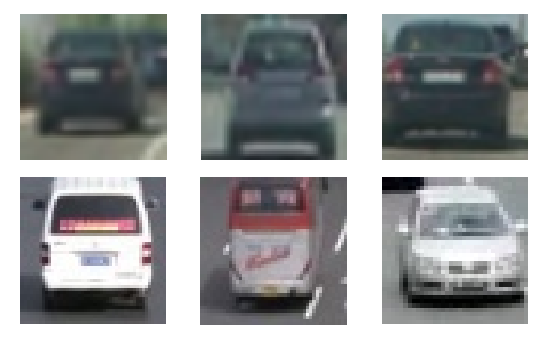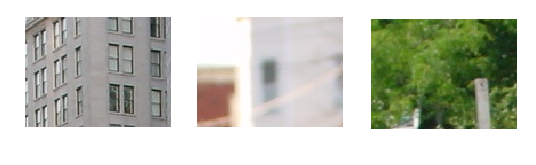• block大小：16x16
• window大小：64x64
• cell大小：4x4
• block步长：x方向为8，y方向为8
• window步长：x方向为8，y方向为8

$dimension=9\ast \frac{bloc{k}_{x}}{cel{l}_{x}}\ast \frac{bloc{k}_{y}}{cel{l}_{y}}\ast \left(1+\frac{windo{w}_{x}-bloc{k}_{x}}{blockstrid{e}_{x}}\right)\ast \left(1+\frac{windo{w}_{y}-bloc{k}_{y}}{blockstrid{e}_{y}}\right)$

Mat GetHOGfeature(string imgname){
resize(img, img, Size(Imgheight, Imgwidth));
Ptr<HOGDescriptor> hog = new HOGDescriptor(Size(Window_y, Window_x),
Size(block_y, block_x),
Size(block_stride_y, block_stride_x),
Size(cell_y, cell_x), 9);
assert(hog->getDescriptorSize() == dimension);
vector<float> descriptor;
hog->compute(img, descriptor, Size(Window_stride_y, Window_stride_x), Size(0, 0));
assert(descriptor.size() == dimension);
Mat s(descriptor);
transpose(s, s);
return s;
}

void HOGSVMtrainAuto(string trainlist){
Mat Data4Train(0, dimension, CV_32FC1), labels(0, 1, CV_32SC1);
GetAllImgHOGfeature(Data4Train, labels, trainlist, ImgTrainPath);

struct timeval pre, after;
gettimeofday(&pre, NULL);

Ptr<ml::SVM> model = ml::SVM::create();
model->setKernel(ml::SVM::KernelTypes::LINEAR);
model->setType(ml::SVM::C_SVC);
model->setP(1e-2);
model->setC(1);
model->setGamma(1e-2);
model->setTermCriteria(cvTermCriteria(CV_TERMCRIT_ITER, 10000, 0.000001));

if(debug){
cout << "height: "<<Data4Train.rows << ", width: " << Data4Train.cols << endl;
cout << "trainingdata depth: " << Data4Train.depth() << endl;
cout << "label depth: " << labels.depth() << endl;
cout << "trainingdata type " << Data4Train.type() << endl;
cout << "label type " << labels.type() << endl;
}

assert(Data4Train.type() == CV_32FC1);
assert(labels.type() == CV_32SC1);

Ptr<ml::TrainData> data = ml::TrainData::create(Data4Train, ml::ROW_SAMPLE, labels);
cout << "start training ..." << endl;
model->trainAuto(data, 10);
cout << "finish training ..." << endl;
gettimeofday(&after, NULL);
cout << "training time: " << after.tv_sec - pre.tv_sec << "s"<< endl;
model->save("../model/svm_hog_classifier.xml");
cout << "model saving fininshed ..." << endl;
}

#### 根据haar特征进行训练

Haar-like特征点，是一种简单的特征描述，其理论相当容易理解，就是按照下图的模式来计算白色窗口的像素总和和黑色窗口像素总和的差，如下图：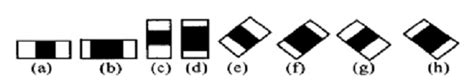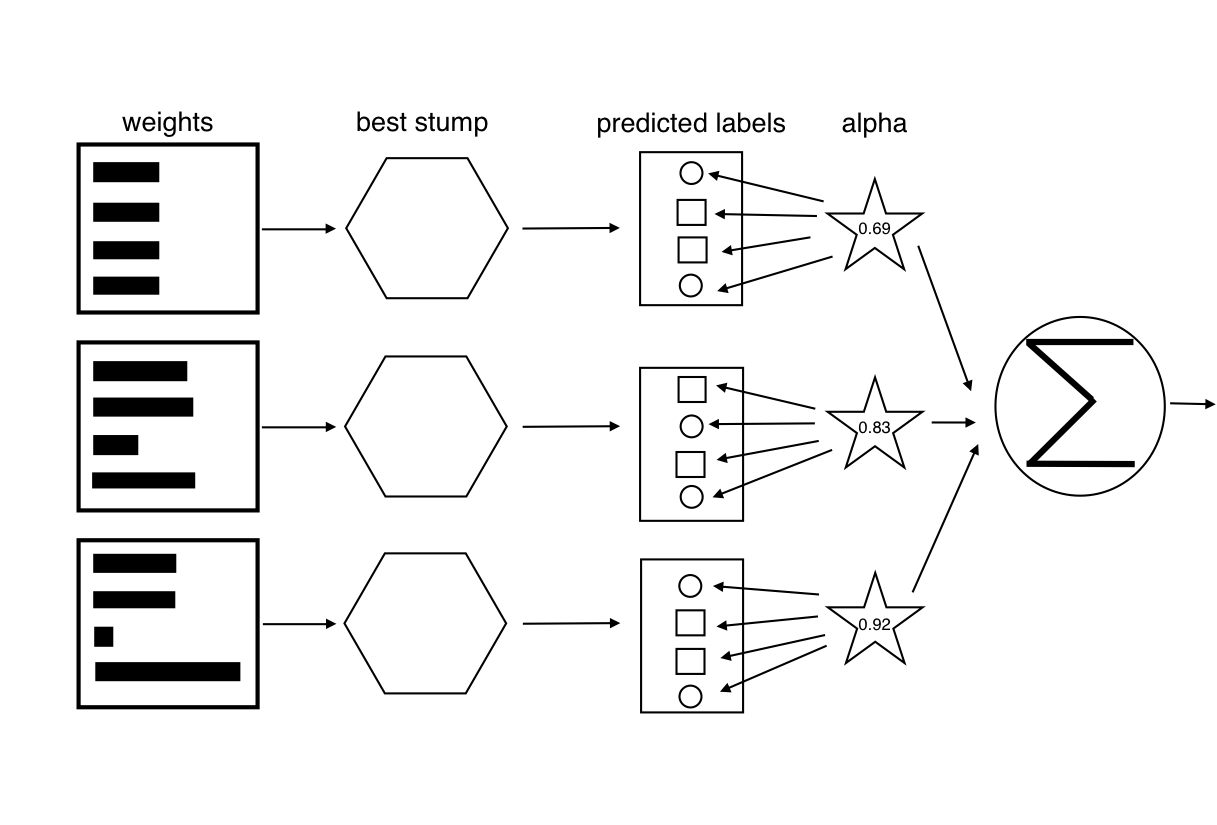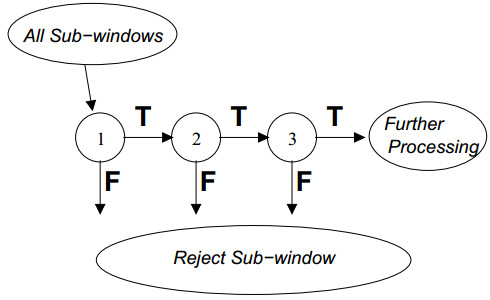../../../data/ProData3/17622107.jpg 1 0 0 38 38
../../../data/ProData3/112237754.BMP 1 0 0 128 96
../../../data/ProData3/12130486.BMP 1 0 0 128 96
...

../../../data/ProData4/174157305.jpg
../../../data/ProData4/18635891.jpg
../../../data/ProData4/1356388.jpg
...

opencv提供了opencv_createsamples.exe建立训练所需要的参数列表，在命令行中调用该exe，输入如下命令：

opencv_createsamples -vec pos.vec -info pos_info.dat -bg neg_info.dat -num 2000 -w 24 -b 24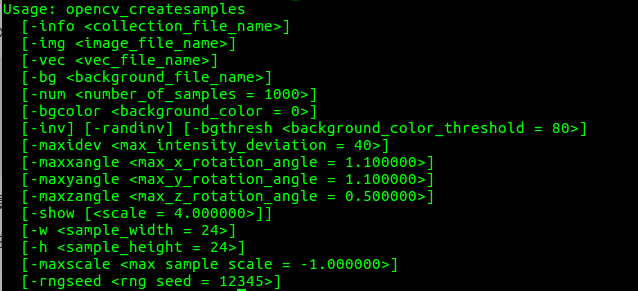opencv_traincascade -data ../model/adaboost -vec pos.vec -bg neg_info.dat -numPos 2000 -numNeg 7000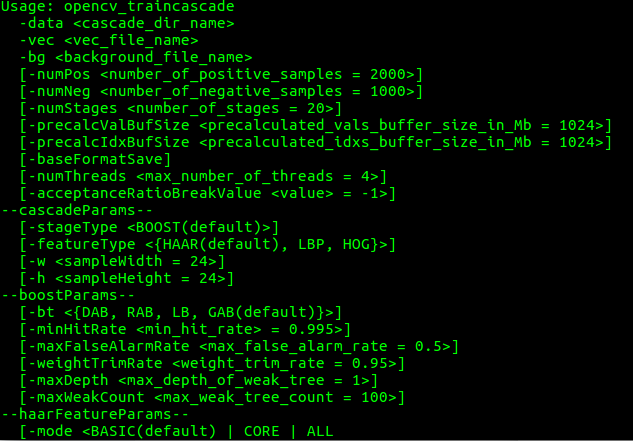#### 最终检测

• 对图像进行缩放，resize到448x448
• 滑动窗口以一定比例放大，对图像进行多尺度检测，避免漏检较大的车辆
• 对所有结果进行非极大值抑制，得出最终检测结果

void FinalDetect(string filename, string model_cascade, string model_hog, int dataset = 1, bool IsLine = false) {
setUseOptimized(true);

HOGDescriptor my_hog(Size(Window_y, Window_x), Size(block_y, block_x), Size(block_stride_y, block_stride_x),
Size(cell_y, cell_x), 9);
//get support vector from model
Mat sv = model->getSupportVectors();
vector<float> hog_detector;
const int sv_total = sv.cols;
Mat alpha, svidx;
double rho = model->getDecisionFunction(0, alpha, svidx);
Mat alpha2;
alpha.convertTo(alpha2, CV_32FC1);
Mat result(1, sv_total, CV_32FC1);
result = alpha2 * sv;
for (int i = 0; i < sv_total; ++i)
hog_detector.push_back(-1 * result.at<float>(0, i));
hog_detector.push_back((float) rho);
my_hog.setSVMDetector(hog_detector);

vector<Rect> detections;
vector<double> foundWeights;
vector<int> rejLevel;
vector<bbox_info> dets;
vector<bbox_info> keep;
VideoCapture cap;
cap.open(filename);
while (true) {
Mat img;
cap >> img;
if (!img.data)
break;
resize(img, img, Size(448, 448));
cout << img.size() << endl;
if (IsLine)
LineDetect2(img, dataset);

detections.clear();
foundWeights.clear();
rejLevel.clear();
dets.clear();
keep.clear();

my_hog.detectMultiScale(img, detections, foundWeights, 0, Size(8, 8), Size(), 1.1, 2., true);
cout << "hog detect object: " << detections.size() << endl;
for (size_t i = 0; i < detections.size(); i++) {
if (foundWeights[i] > 1.3) {
bbox_info tmp_bbox(detections[i].x, detections[i].y, detections[i].br().x, detections[i].br().y,
foundWeights[i]);
dets.push_back(tmp_bbox);
}
}

car_classifier.detectMultiScale(img, detections, rejLevel, foundWeights, 1.1, 3, 0, Size(), Size(), true);
cout << "cascade detect object: " << detections.size() << endl;
for (int i = 0; i < detections.size(); i++) {
if (rejLevel[i] < 20 || foundWeights[i] < 1.)
continue;
bbox_info tmp(detections[i].x, detections[i].y, detections[i].br().x, detections[i].br().y,
foundWeights[i]);
dets.push_back(tmp);
}

keep = nms(dets);
for (size_t i = 0; i < keep.size(); i++) {
Point p1(keep[i].xmin, keep[i].ymin), p2(keep[i].xmax, keep[i].ymax);
Scalar color(0, 255, 0);
rectangle(img, p1, p2, color, 2);
}
imshow("detect", img);
waitKey(0);

}
}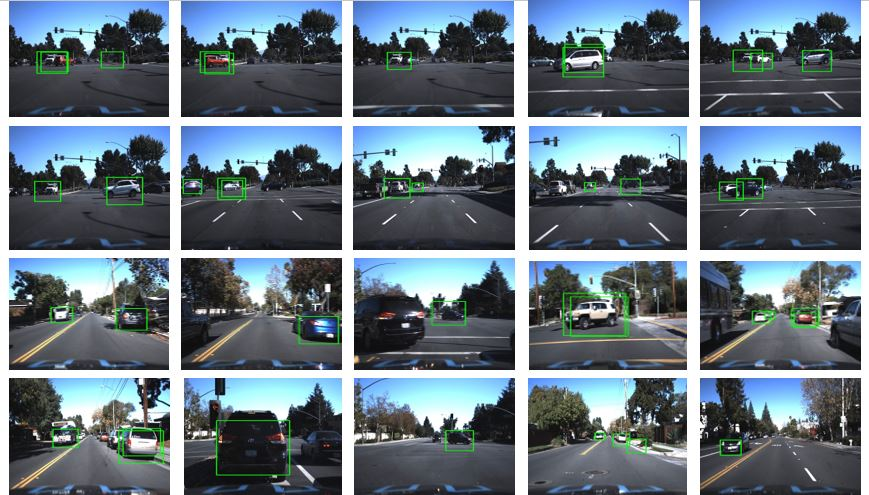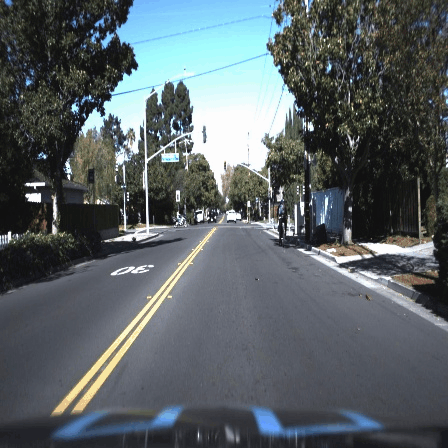### 直线检测

1. 对图像进行透视变换，使其变为鸟瞰图：
Point2f origin[] = {Point2f(204, 286), Point2f(71, 448), Point2f(394, 448), Point2f(243, 286)};
Point2f dst[] = {Point2f(112, 0), Point2f(112, 448), Point2f(336, 448), Point2f(336, 0)};
trans = getPerspectiveTransform(origin, dst);
warpPerspective(img_o ,img, trans, img.size());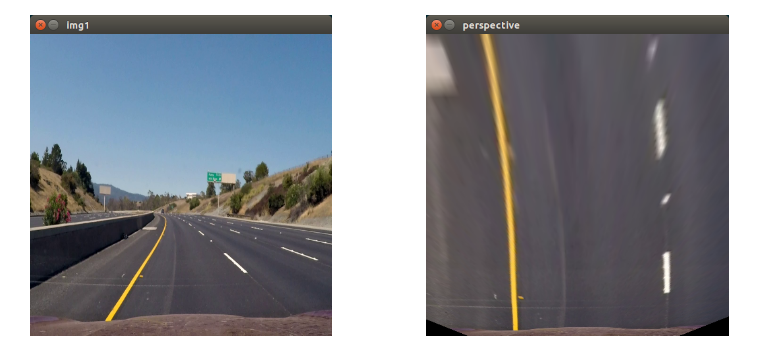1. 对原图像进行x-sobel滤波，并进行阈值过滤
void mag_threshold(const Mat img, Mat &out, int sobel_kernel, int min_thres, int max_thres) {

cvtColor(img, out, CV_BGR2GRAY);
Sobel(out, out, CV_8UC1, 1, 0, sobel_kernel);
normalize(out, out, 0, 255, NORM_MINMAX);
threshold(out, out, min_thres, 0, THRESH_TOZERO);
threshold(out, out, max_thres, 255, THRESH_BINARY);
}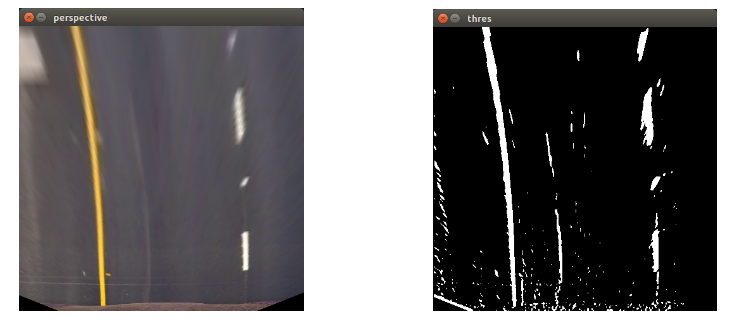1. 对原图像转换到HLS空间，保留黄色和白色(车道多为黄色和白色)
void yellow_white_threshold(Mat origin, Mat &out1) {
int y_lower[] = {10, 0, 100};
int y_upper[] = {40, 255, 255};
int w_lower[] = {0, 200, 0};
int w_upper[] = {180, 255, 255};
cvtColor(origin, HLS, CV_BGR2HLS);

vector<int> yellow_lower(y_lower, y_lower + 3);
vector<int> yellow_upper(y_upper, y_upper + 3);
vector<int> white_lower(w_lower, w_lower + 3);
vector<int> white_upper(w_upper, w_upper + 3);

cvtColor(out1, out1, CV_HLS2BGR);
cvtColor(out1, out1, CV_BGR2GRAY);
threshold(out1, out1, 130, 255, THRESH_BINARY);
}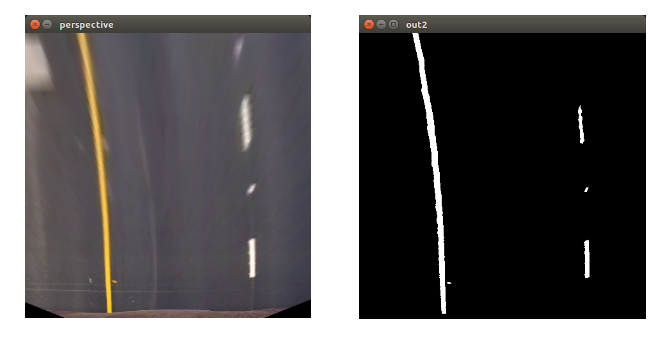1. 根据2,3步得到最终的二值图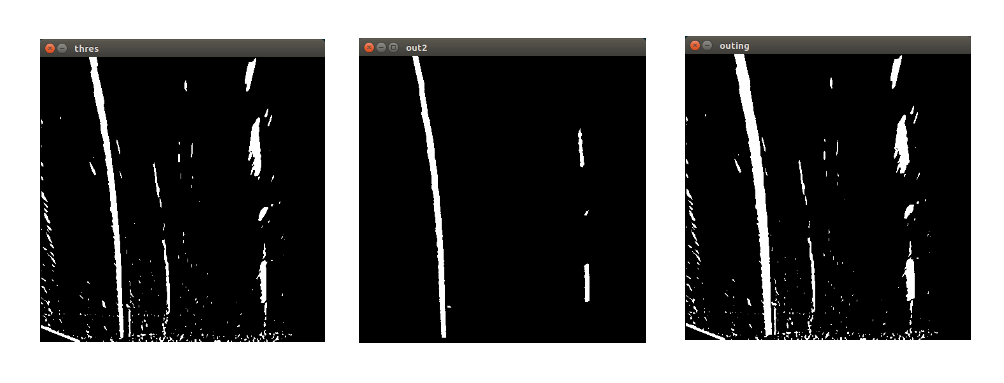1. 利用霍夫变换找出相应的直线端点(根据直线斜率进行一定的限制)
    vector<Vec4i> lines;
vector<Point2f> leftlines;
vector<Point2f> rightlines;
HoughLinesP(out1, lines, 1, CV_PI / 180, 50, 30, 10);
cout << lines.size() << endl;
for (size_t i = 0; i < lines.size(); i++) {
//abandon horizontal line.
if (lines[i] == lines[i])
continue;
//get left lines
if (lines[i] <= 224 && lines[i] <=224){
float k = 1.5;
//if not verticle line
if (lines[i] != lines[i])
k = fabs(float(lines[i]-lines[i])/float(lines[i]-lines[i]));
if (k>=1.5) {
leftlines.push_back(Point2f(lines[i], lines[i]));
leftlines.push_back(Point2f(lines[i], lines[i]));
}

}
1. 对这些点进行线性回归
    Vec4f line_left, line_right;
fitLine(leftlines, line_left, DIST_L1, 0, 0.01, 0.01);
fitLine(rightlines, line_right, DIST_L1, 0, 0.01, 0.01);
1. 画出直线围成的区域，并进行高亮，显示到原图上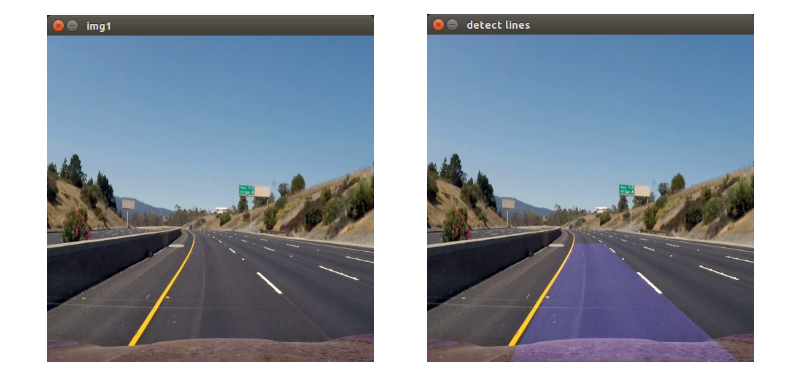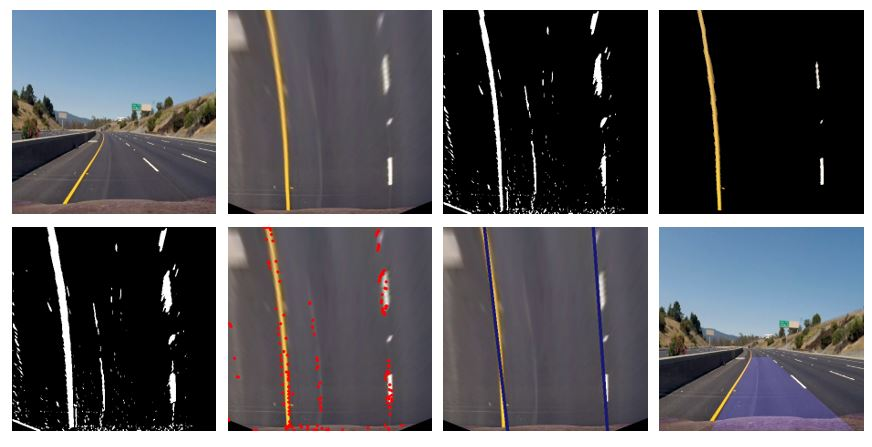639 人正在学习 去看看 白勇

# 无人驾驶汽车系统入门（六）——基于传统计算机视觉的车道线检测(1)

## 视觉感知VS激光雷达## 相机标定1. 切向畸变（tangential distortion）：是由于透镜本身与相机传感器平面（成像平面）或图像平面不平行而产生的，这种情况多是由于透镜被粘贴到镜头模组上的安装偏差导致。

xcorr=xdis(1+k1r2+k2r4+k3r6)

ycorr=ydis(1+k1r2+k2r4+k3r6)

xcorr=xdis+[2p1xdisydis+p2(r2+2x2dis)]

ycorr=ydis+[p1(r2+2y2dis)+2p2xdisydis]

• xdis$x_{dis}$ydis$y_{dis}$ 表示有畸变的坐标；
• xcorr$x_{corr}$ycorr$y_{corr}$ 表示修复后的坐标；
• k1$k_1$k2$k_2$k3$k_3$ 表示径向畸变参数；
• p1$p_1$p2$p_2$ 表示切向畸变参数；
• r$r$ 表示矫正以后的坐标到图片中心的距离#### 相机，棋盘图#### 使用OpenCV找出棋盘的对角点

from __future__ import print_function

import numpy as np
import cv2
import matplotlib.pyplot as plt
import pickle
%matplotlib inline

fig1 = plt.figure(1, figsize=(16, 9))
cal_gray = cv2.cvtColor(cal, cv2.COLOR_RGB2GRAY)
plt.subplot(2,2,1)
plt.imshow(cal)
plt.subplot(2,2,2)
plt.imshow(cal_gray, cmap='gray')ret, corners = cv2.findChessboardCorners(cal_gray, (9, 6),None)
if ret == True:
cal = cv2.drawChessboardCorners(cal, (9, 6), corners, ret)
plt.imshow(cal)objp = np.zeros((6*9, 3), np.float32)
objp[:, :2] = np.mgrid[0:9, 0:6].T.reshape(-1, 2)

img_points = []
obj_points = []

img_points.append(corners)
obj_points.append(objp)

image_size = (cal.shape, cal.shape)
ret, mtx, dist, rvecs, tvecs = cv2.calibrateCamera(obj_points, img_points,
image_size, None, None)

# Read in a test image
undist = cv2.undistort(img, mtx, dist, None, mtx)
plt.subplot(2,2,1)
plt.imshow(img)
plt.subplot(2,2,2)
plt.imshow(undist)## 确定ROI

ROI(Region of interest) 即我们处理一个视觉任务时“感兴趣的区域”，当然不同的任务ROI是不一样的，对于车道线检测而言（如下图），ROI就是车辆的前方的车道线区域：def perspective_transform(img, M):
img_size = (img.shape, img.shape)
warped = cv2.warpPerspective(img, M, img_size, flags=cv2.INTER_LINEAR)
return warped

# left_top to left_bottom,
corners = [(603, 445), (677, 445), (1105, 720), (205, 720)]

wrap_offset = 150
src_corners = [(603, 445), (677, 445), (1105, 720), (205, 720)]
dst_corners = [(205 + wrap_offset, 0), (1105 - wrap_offset, 0), (1105 - wrap_offset, 720), (205 + wrap_offset, 720)]
M = cv2.getPerspectiveTransform(np.float32(src_corners), np.float32(dst_corners))
wrap_img= perspective_transform(straight_lines1, M)

subplot(1, 2, [straight_lines1, wrap_img])2018-12-20 16:21:43 sru_alo 阅读数 398

639 人正在学习 去看看 白勇

最近要做研究生计算机视觉课程的大作业，正好了解到Udacity有一个《无人驾驶车工程师》的课程，其中有一个高级车道线检测的项目，所以就准备以此为课题完成作业，并且学习一下高级车道线检测。关于车道线检测的相关资料还是很多的，我找到了一个感觉效果不错然后相关讲解还算细致的项目，准备入手。

1. 相机图像校准算法。主要用于纠正经过相机镜头成像后图片四边的变形或者拍摄物体与相机角度不平行、不正对；

2. 车道线透视图角度的拉平，转换成鸟瞰图下的平行线；

3. 用多种Sobel梯度过滤算法，如x轴方向、正切方向、向量值大小来滤除噪声；

4. 直方图峰值法寻找窄窗内的白线

5. 二阶曲线拟合确定相关系数，计算曲率和弯道半径

2012-04-16 13:53:24 abcjennifer 阅读数 5443

639 人正在学习 去看看 白勇

===========================Feature Extraction===========================

1.基于光强梯度提取2. 基于车道宽度提取

3.选取一个线段的重点为feature point

============================Matching============================

============================Tracking============================

Define:

Ali & Arias left and right accumulator respectively，一旦其超过阈值，以后就都由另一侧的车道预测左侧车道位置

Amaxas the threshold of Ali & Ari

L as slide window size

[u,v] as 第t-1帧中scan line u 上的feature point 坐标

Initialize Ali=Ari=1;
Search 在第t帧中[u,v-L] 到[u,v+L]区间内是否有feature point
if(exist)
取一个离[u,v]最近的点作为t帧的跟踪点，Ali=1;
else
if(Ali<Amax)
第t帧的feature point=第t-1帧的feature point，Ali++;
else if(Ari<Amax)
第t帧的feature point由右车道点-车道宽度给出
else
Adjust Parameters and resume from feature extraction